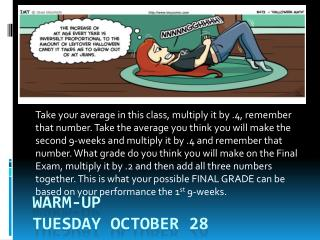DownloadDownload PresentationWarm-Up Tuesday October 28

Warm-Up Tuesday October 28

Download PresentationWarm-Up Tuesday October 28

- - - - - - - - - - - - - - - - - - - - - - - - - - - E N D - - - - - - - - - - - - - - - - - - - - - - - - - - -
Presentation Transcript

1. Take your average in this class, multiply it by .4, remember that number. Take the average you think you will make the second 9-weeks and multiply it by .4 and remember that number. What grade do you think you will make on the Final Exam, multiply it by .2 and then add all three numbers together. This is what your possible FINAL GRADE can be based on your performance the 1st 9-weeks. Warm-UpTuesday October 28

2. How would you solve this problem? The school that Alberto goes to is selling tickets to the annual talent show. On the first day of ticket sales the school sold 3 adult tickets and 7 child tickets for a total of \$63. The school took in \$77 on the second day by selling 5 adult tickets and 7 child tickets. Find the price of an adult ticket and the price of a child ticket.

3. So there are multiple ways to solve this problem. But all involve equations Systems of Equations to be more precise They can be solved algebraically.

4. First lets review how to solve algebraically Substitution!!

5. Solve the following system by substitution. 2x – 3y = –24x +   y = 24 Solvetheeasierequationforthe variable with a coefficient of 1 ifthereisone. y = –4x + 24 Now I'll plug this in ("substitute it") for "y" in the first equation, and solve for x 2x – 3(–4x + 24) = –22x + 12x – 72 = –214x = 70x = 5

6. Now I can plug this x-value back into either equation, and solve for y. But since I already have an expression for "y =", it will be simplest to just plug into this: y = –4(5) + 24 = –20 + 24 = 4 Then the solution is (x, y) = (5, 4).

7. Solve the following system by substitution.     7x + 2y = 16–21x – 6y = 24 Neitherequationiseasytosolvefor a variable so just pick eitheronetosolvefor. 7x+ 2y = 162y = –7x + 16y = –( 7/2 )x + 8 Now I'll plug this into the other equation –21x – 6(–( 7/2 )x + 8) = 24–21x + 21x – 48 = 24–48 = 24 Ummmm.NO!

8. Classwork/Homework Complete all problems on the Solving systems by Substitution worksheet, the word problems as well!